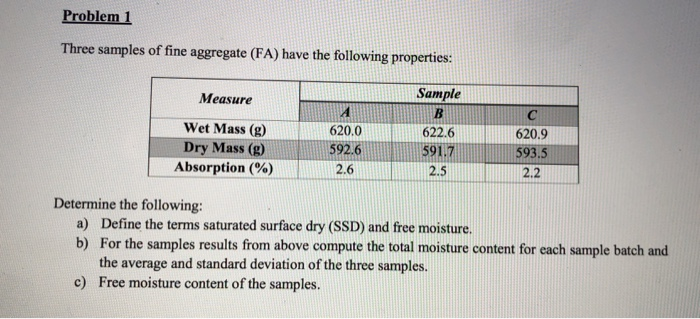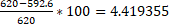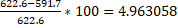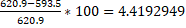Homework Help Question & Answers

# Problem 1 Three samples of fine aggregate (FA) have the following properties Sample Measure Wet Mass (g) Dry Mass (g) Absorption (%) 622.6 591.7 620.9 593 620.0 592.6 2.6 2.52.2 Determine the fol...Problem 1 Three samples of fine aggregate (FA) have the following properties Sample Measure Wet Mass (g) Dry Mass (g) Absorption (%) 622.6 591.7 620.9 593 620.0 592.6 2.6 2.52.2 Determine the following: a) Define the terms saturated surface dry (SSD) and free moisture. b) For the samples results from above compute the total moisture content for each sample batch and the average and standard deviation of the three samples. Free moisture content of the samples. c)

Saturated surface dry:

It is defined as the condition in aggregates in which the surface is dry but the inner particle void are saturated with water, this condition make no surface adsorption through aggregates.

Free moisture:

It is defined as the moisture not retained or absorbed by the aggregates, also the moisture which can be removed by air drying.

TOTAL MOISTURE CONTENT-

Sample A = 620-592.6 = 27.4g

Sample B = 622.6-591.7 = 30.9g

Sample C= 620.9-593.5 = 27.4g

AVERAGE

FOR WET SAMPLES A, B, & C = 621.1667

FOR DRY SAMPLES A, B, & C = 592.6

STANDARD DEVIATION

FOR WET SAMPLES A, B, & C = 1.320353

FOR DRY SAMPLES A, B, & C = 0.9

FREE MOISTURE CONTENT

SAMPLE A =SAMPLE B =SAMPLE C =##### Add Answer of: Problem 1 Three samples of fine aggregate (FA) have the following properties Sample Measure Wet Mass (g) Dry Mass (g) Absorption (%) 622.6 591.7 620.9 593 620.0 592.6 2.6 2.52.2 Determine the fol...
More Homework Help Questions Additional questions in this topic.

• #### Would my calculated molarity of HOAc in vinegar be too high, low, or unchanged if a) i forgot to rinse wet buret with vinegar b)delivered vinegar into a wet flask c)used a buret with a broken tip Any help please

Need Online Homework Help?

Get FREE EXPERT Answers
WITHIN MINUTES
Related Questions